﻿
It is the top of the page.

#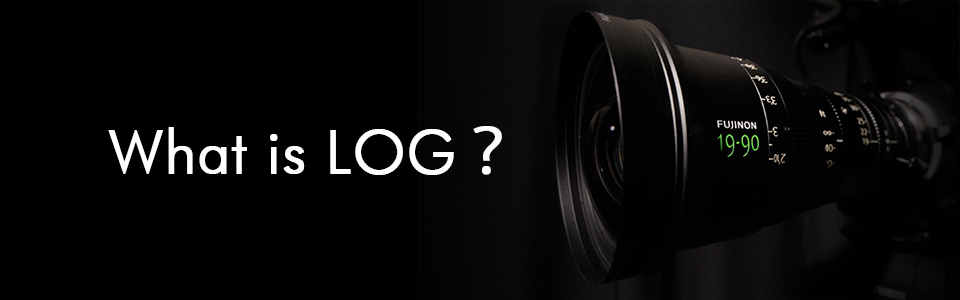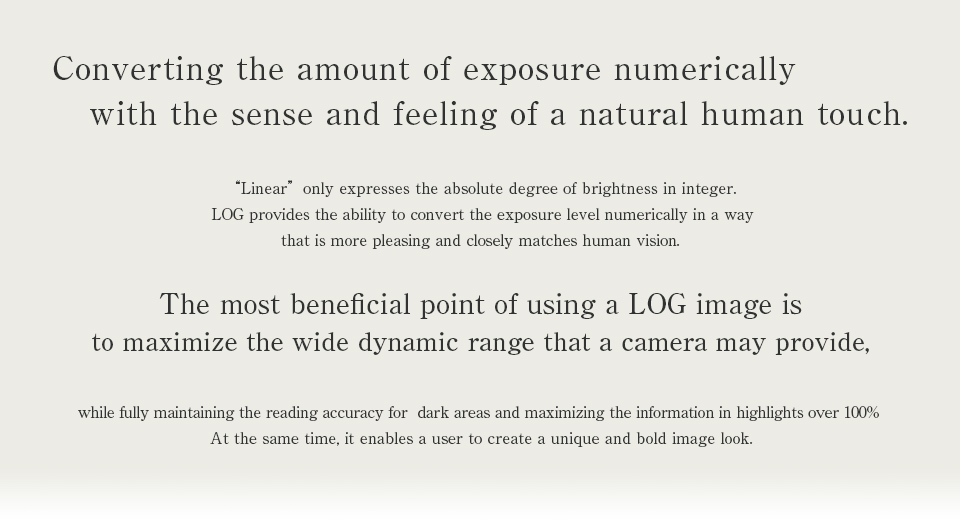##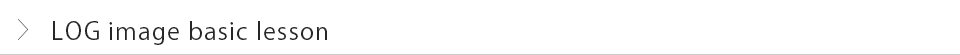### Hi, everybody. This is Uchida from TVLogic. On the following pages, I would like to provide some lessons about motion picture production using LOG images. These are some of the topics:

• First of the all, what is a LOG image?

• I want to produce motion pictures using LOG images, but do can I do it?

• I am producing motion pictures using LOG images, but how can I improve the efficiency and quality of the process?

##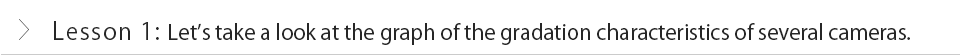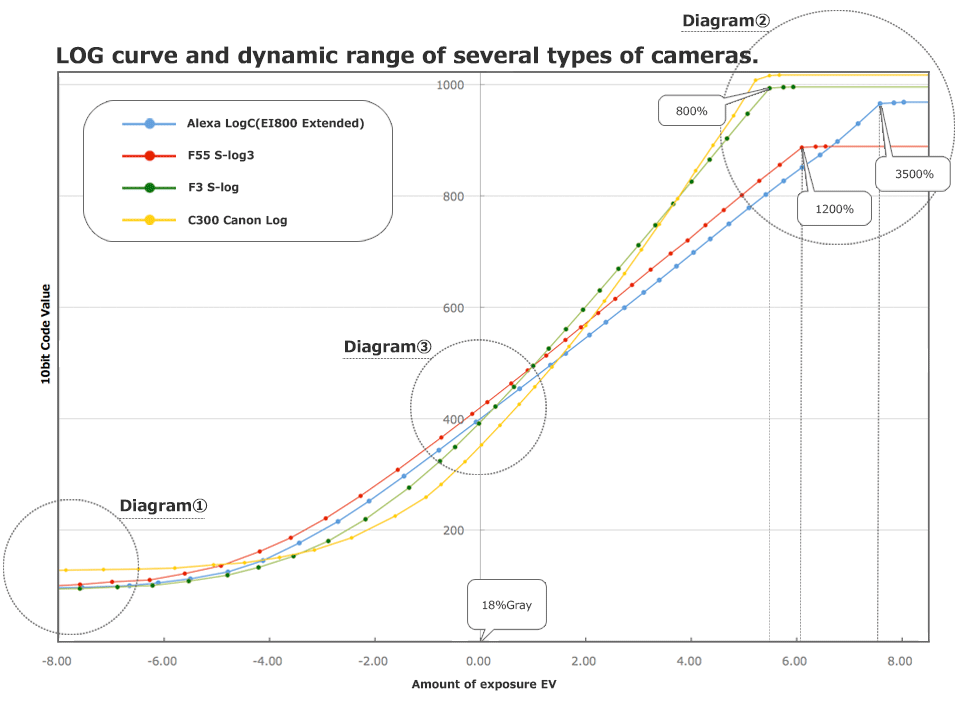### To start, I would like to explain briefly how to read the gradation characteristics graph of each camera. The y-axis represents 10bit-code values (CV), and x-axis represents the exposure amount (logarithmic scale), respectively. Unlike conventional film cinema camera recording, digital cinema cameras convert and record the light into electrical signals through an image sensor. The y-axis 10bit-CV expresses the amount of light as 10bit, which equals to 210=1024 as an integer. Namely, 101 is the next whole number to 100, in other words it cannot be 100.5. Therefore, the number between 100.01 and 100.99 will be recorded as 100 or 101. The x-axis represents the exposure amount in logarithm scale: 0 of the x value is adjusted at 18% gray. As x value increases by 1 in regards to the aperture. (1 step up = the amount of exposure will be doubled) Clearly, as the value increases, it gets brighter. The graph above illustrates the brightness up to around 5000% that is equal to 50 times brighter than 100% white. The region drawn as a linear slope line is a region where the camera can record the brightness of the subject. Also, this region represents each camera's dynamic range. Do you notice something by looking at this graph?

• Pure black is not represented as zero. Although the adjusted value varies with each camera maker, an offset value is added. (Diagram ①)

※ Pure black means the measured value of original scene that has no light.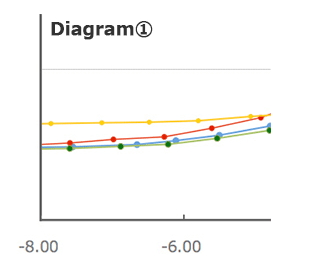Offset value is added; therefore, the value cannot be 0.

• The maximum cv is not always equal to 10-bit maximum 1023.

Some cameras reach the maximum (converge) before 1023. (Diagram ②)

• It varies from camera to camera model on how far they record brightness (highlight side of dynamic range). (Diagram ②)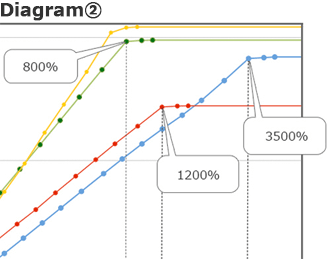CV reaches the maximum before 1023.
Also, the maximum exposure amount varies on cameras.

• CV of 18% gray varies on cameras.

Therefore, the appropriate exposure amount cannot be defined in a single point. (Diagram ③)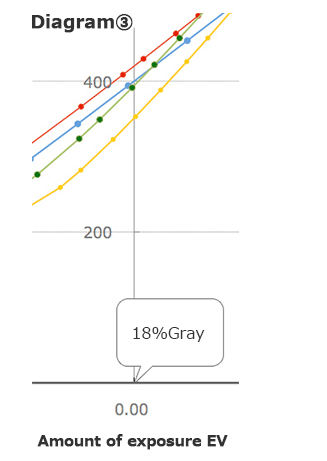• There will be various slopes with halftone depending on the camera type; however each camera type has almost a linear area. (This is why we call it LOG).

### Mr. Uchida's profileHe refers to himself as an expert problem solver in image system designs. Starting his career with consumer photos, Mr. Uchida was involved in designing systems using LOG images since 1995 predating the release of Cineon. Currently, he devotes himself enthusiastically to creating solutions which maximize a camera's full feature set with ease.

From here to the footer.